Select Page

# Maths 12 Science CBSE Probability Answers for MCQ in English

Maths 12 Science CBSE Probability Answers for MCQ in English to enable students to get Answers in a narrative video format for the specific question.

Expert Teacher provides Maths 12 Science CBSE Probability Answers for MCQ through Video Answers in English language. This video solution will be useful for students to understand how to write an answer in exam in order to score more marks. This teacher uses a narrative style for a question from Probability not only to explain the proper method of answering question, but deriving right answer too.

Please find the question below and view the Answer in a narrative video format.

Question:

## Similar Questions from CBSE, 12th Science, Maths, Probability

Question 1 : In a group of 30 scientists workings on an experiment, 20 never commit error in their work and are reporting results elaborately. Two scientists are selected at random from the group. Find the probability distribution of the number of selected scientists who never commit error in work and reporting. Also find the mean of the distribution. What values are described in this question?  (View Answer Video)

Question 2 : Five bad oranges are accidently mixed with 20 good ones. If four oranges are drawn one by one successively with replacement, then find the probability distribution of number of bad oranges drawn. Hence, find the mean and variance of the distribution.    (View Answer Video)

Question 3 :  A bag contains 3 red and 7 black balls. 2 balls are selected at random one by one without replacement. If the second selected ball happens to be red, what is the probability that the first selected ball is also red?   (View Answer Video)

Question 4 : A box contains 50 bolts and 50 nuts. Half of the bolts and nuts are rusted. If two items are drawn with replacement, what is the probability that either both are rusted or both are bolts.    (View Answer Video)

Question 5 : One card is drawn from a pack of 52 cards. Find the probability of getting a black face card: (View Answer Video)

### Three Dimensional Geometry

Question 1 : Find the angle between the planes 7x + 2y + 6z = 15 and 3x - y + 10z = 17. (View Answer Video)

Question 3 : Find the equation of the plane passing through the line of intersection of the planes x + y + z = 1 and 2x + 3y + 4z = 5 which is perpendicular to the plane x - y + z = 0. Also, find the distance of the plane obtained above from the origin. (View Answer Video)

Question 4 : Find the angle between the lines whose direction ratios are a, b, c and b - c, c - a, a - b. (View Answer Video)

Question 5 : Find the distance of the point (1, -2, 3) from the plane x-y+z=5 measured parallel to the line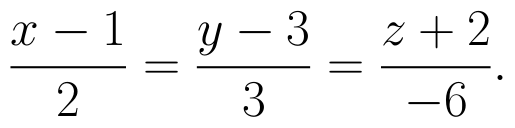(View Answer Video)

### Application of Integrals

Question 1 : Using the method of integration, find the area of the region bounded by the lines: 5x - 2y -10 = 0, x + y - 9 = 0 and 2x - 5y - 4 = 0. (View Answer Video)

Question 2 : Find the area of the given curves and given lines: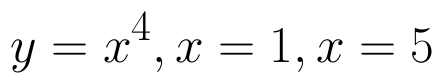and x-axis (View Answer Video)

Question 3 : Find the area of smaller region bounded by the ellipse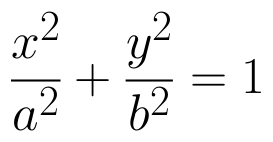and the line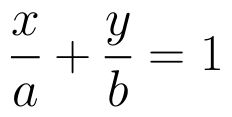. (View Answer Video)

Question 4 : Find the area of the region enclosed by the parabola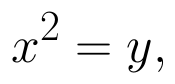the line y = x + 2. (View Answer Video)

Question 5 : Using the method of integration, find the area of the region bounded by the lines 3x - 2y + 1 = 0, 2x + 3y -21 = 0 and x - 5y + 9 = 0. (View Answer Video)

### Continuity and Differentiability

Question 1 : Differentiate w.r.t.x the function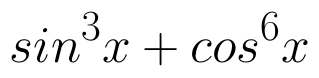. (View Answer Video)

Question 2 : If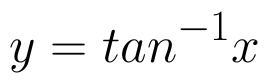, find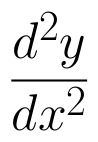in terms of y alone. (View Answer Video)

Question 3 :  Find the second order derivative of the function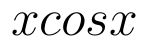. (View Answer Video)

Question 4 :  Find the second order derivative of the function. (View Answer Video)

Question 5 : Differentiate w.r.t.x the function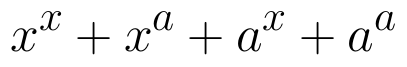, for some fixed a > 0 and x > 0. (View Answer Video)